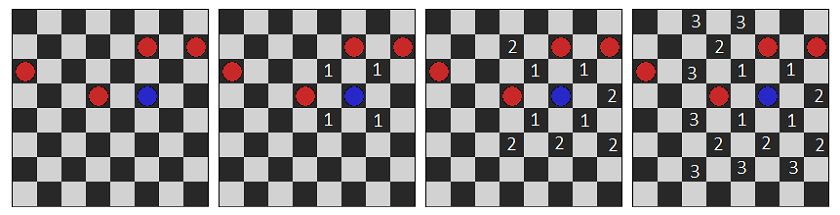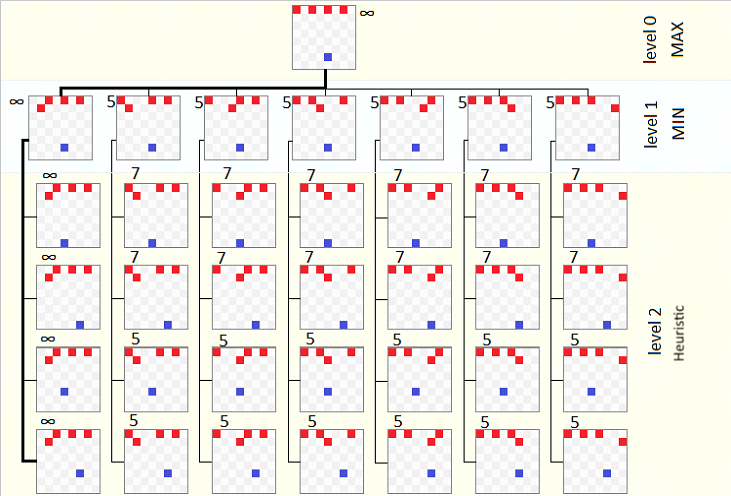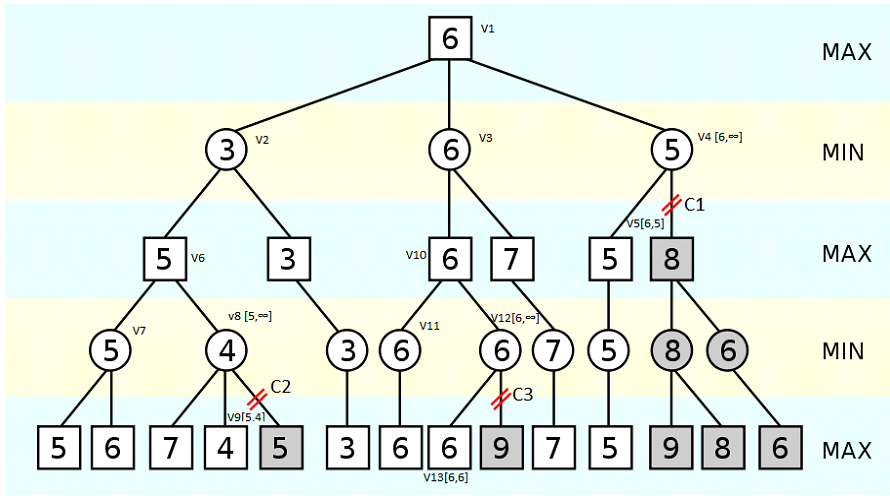# Minimax Alpha Beta Pruning Sample

This article is intended to explain the essence of the fundamental methods of construction and optimization of artificial intelligence for computer games (mainly antagonistic). For example, “Minimax” algorithm and it’s “alpha-beta pruning” optimizations in the Rabbits&Wolves game.

There are 4 wolves at the top of a chessboard (in black cells), and 1 rabbit at the bottom (on one of the black cells). The rabbit goes first. He can walk only one cell at a diagonal, though wolves can only go down. Rabbit wins when he reaches one of the top cells of the chessboard, and the wolves win when they surround and catch the rabbit (when he has no place to go).

Heuristic
In practical engineering sense, minimax method is based on heuristics that we will consider before proceeding to the core algorithms.

In the context of minimax, heuristic is needed to estimate the probability of victory of one of players, for any state. The challenge is to build a heuristic evaluation function that quickly and accurately can indicate the probability of victory of a particular player for a particular arrangement of figures, not considering the way the players have come to this state. In my example, the evaluation function returns a value from 0 to 254, where 0 means victory of the rabbit, 254 victory of the wolf, intermediate values mean the interpolation of the two estimates.

An example of the evaluation function

• Hair has as much chances to win, as close he is to the top of the chessboard. This heuristic is effective in terms of performance (O (1)), but it is not suitable algorithmically. Rabbit’s “height” correlates with the probability of winning, but it distorts the main purpose of a rabbit – to win. This evaluation function says the rabbit to move up, but leads to the fact that the rabbit moves up, in spite of the obstacles, and falls into the trap.
• Rabbit has as much chances to win, the less moves he has to make to win, assuming the wolves are frozen. It is more cumbersome algorithm with complexity O(n), where n is a number of cells. Calculating the number of moves requires breadth-first search:|The code to do breadth-first search (filling the map with values equal to “distance” from the rabbit):

```this->queue.push(this->rabbitPosition);
while (!this->queue.empty())
{
Point pos = this->queue.pop();
for (int i = 0; i < 4; i++) if (canMove(pos + this->possibleMoves[i]))
{
Point n = pos + this->possibleMoves[i];
this->map[n.y()][n.x()] = this->map[pos.y()][pos.x()] + 1;
this->queue.push(n);
}
}
```

The result of the evaluation function must be a value of “Distance” to the nearest upper cells, or 254 if it is impossible to reach them. Despite the shortcomings that I will describe below, this is the heuristics used in the game.

Minimax

Some concepts and notation:
State – a location of the pieces on the board.
Terminal state – a condition from which there is no more moves (someone wins / draw).
Vi – i-th state.
Vik – k-th state which you can reach in one move from a state of Vi.
f(Vi) – estimate of the probability of victory for the state of Vi.
g(Vi) – heuristic evaluation of winning probability for the state Vi.

The method was developed to solve the problem of choosing a course for Vi state. It is logical to choose the course for which the estimate of the probability will be the most advantageous for a player who goes. Our metric for wolves is the maximum score for hare minimum. A score is calculated as follows:

f(Vi) = g(Vi), if Vi is a terminal state or limit of settlements depth is reached.
f(Vi) = max (f(Vi1), f(Vi2) … f(Vik)), if Vi is a state of the player who is looking for the maximum assessment.
f(Vi) = min (f(Vi1), f(Vi2) … f (Vik)), if Vi is a state of the player who is looking for the minimum assessment.

The method is based on common sense. We make our move to maximize our assessment of the victory, but to calculate anything, we need to know how the enemy will go, and the enemy is going to maximize the evaluation of his victory. In other words, we know how the enemy will go, and thus can build probability assessment.

Example:In this example, the player plays for the rabbit and the computer for the wolves.
The algorithm iterates through all possible moves for a wolf and assesses the probability of winning.

This example will be easier to understand if we consider it from the bottom. At the second level, we have the state, for which we have a heuristic evaluation. On the second level, the first one gets its scores, respectively, by the minimum values of those checked at the next level. And the zero level selects the maximum assessment of those that the first level provided.
Now we need to select the move. Wolf chooses the move that shows the highest estimate; rabbit chooses the on with the lowest. But estimates for different moves can be equal, so for the wolf we take the first move from the list with the maximal assessment, and for the rabbit the last in the list with a minimal estimate.

An example of implementation of the algorithm:

```//return the current state of f(Vi)
int Game::runMinMax(WolfType wolf, int recLevel)
{
int test = NOT_INITIALIZED;

//return the value of heuristics function on the last level of the tree
if (recLevel >= this->AILevel * 2)
return Heuristics();

//index of the chosen way. 0-7 - possible moves for wolves, 8-11 - moves for rabbit
int move = NOT_INITIALIZED;

bool isWolf = (wolf == MT_WOLF);
int MinMax = isWolf ? MIN_VALUE : MAX_VALUE;

//iterate all possible moves for current wolf
for (int i = (isWolf ? 0 : 8); i < (isWolf ? 8 : 12); i++) { int curWolf = isWolf ? i / 2 + 1 : 0; point curWolfPositions = curWolf == 0 ? rabbit : wolves[curWolf - 1]; point curMove = possibleMoves[isWolf ? i % 2 : i % 4]; if (canMove(curWolfPositions + curMove)) { //go, then work with the state temporaryWolfMovement(curWolf, curMove); //testing the move test = runMinMax(isWolf ? MT_RABBIT : MT_WOLF, recLevel + 1); //if it's better then all previous - remember it if ((test > MinMax && wolf == MT_WOLF) ||
(test <= MinMax && wolf == MT_RABBIT))
{
MinMax = test;
move = i;
}

//and reset the current state
temporaryWolfMovement(curWolf, -curMove);
}
}

if (move == NOT_INITIALIZED)
return Heuristics();

//move
if (recLevel == 0 && move != NOT_INITIALIZED)
{
if (wolf == MT_WOLF)
wolves[move / 2] += possibleMoves[move % 2];
else
rabbit += possibleMoves[move % 4];
}
return MinMax;
}
```

Alpha-beta pruning
Alpha-beta pruning is based on the idea that the analysis of some passages can be terminated early, ignoring the result of their testimony.

Roughly speaking, optimization introduces two additional variables alpha and beta, where alpha is the current maximum value, which is less than the maximization (wolf) player will never choose, and beta is the current minimum value, above which the minimization player (rabbit) will never choose. Initially, they are set to -∞, + ∞ respectively, and to the extent of obtaining estimates f (Vi) are modified:

alpha = max (alpha, f (Vi)); to maximize the level.
beta = min (beta, f (Vi)); to minimize the level.

Once the condition alpha > beta will be true, which means the conflict of expectations, we break Vik analysis and return the last received an assessment of the level.Example Legend:

Vi – Search tree nodes decisions
Vi [alpha, beta] – nodes with the specified current values of alpha, beta.
Ci – i–th alpha–beta pruning.
MIN, MAX – levels of the maximization and minimization respectively.

Pruning 1. After the node has been completely processed V1 to V3 node, and its score is obtained f (V3) = 6, the algorithm specifies the alpha value for that node (V1), and transmits its value to the next node – V4 . Now for all child nodes of V4 minimum alpha = 6. After the node has processed V4 version with the V5 node, and received an estimation f(V5) = 5, the algorithm specifies the value of beta for the V4 node. After the upgrade, for the node V4 we have a situation in which the alpha> beta, and therefore other options V4 doesn’t need not be considered. And it does not matter what kind of evaluation will have an off site.

If the value of the clipped site is less or equal to 5 (beta), then for the node V4 will be selected evaluation, that is not greater than 5, and accordingly a variant node V4 will never be selected by V1, because the evaluation of V3 is better.
If the clipped value is greater or equal to 6 (beta + 1), then the node will no longer be considered as a variant because V4 selects V5, since its score lower (better).

Pruning 2 has absolutely identical in structure.
Alpha-beta pruning is a very simple algorithm to implement. Example of modification for minimax algorithm with alpha-beta pruning:

```int Game::minmax(WolfType wolf, int recLevel/*, int alpha, int beta*/)
{
int test = NOT_INITIALIZED;

if (recLevel >= this->AILevel * 2)
return Heuristics();

int move = NOT_INITIALIZED;
bool isWolf = (wolf == MT_WOLF);
int MinMax = isWolf ? MIN_VALUE : MAX_VALUE;

for (int i = (isWolf ? 0 : 8); i < (isWolf ? 8 : 12); i++) { int curWolf = isWolf ? i / 2 + 1 : 0; point curWolfPositions = curWolf == 0 ? this->rabbit : this->wolves[curWolf - 1];
point curMove = this->possibleMoves[isWolf ? i % 2 : i % 4];
if (canMove(curWolfPositions + curMove))
{
temporaryWolfMovement(curWolf, curMove);
test = minmax(isWolf ? MT_RABBIT : MT_WOLF, recLevel + 1, alpha, beta);
temporaryWolfMovement(curWolf, -curMove);

if ((test > MinMax && wolf == MT_WOLF) ||
(test <= MinMax && wolf == MT_RABBIT))
{
MinMax = test;
move = i;
}

/* if (isWolf)
alpha = qMax(alpha, test);
else
beta = qMin(beta, test);
if (beta < alpha) break; */ } } if (move == NOT_INITIALIZED) return Heuristics(); if (recLevel == 0 && move != NOT_INITIALIZED) { if (wolf == MT_WOLF) this->wolves[move / 2] += this->possibleMoves[move % 2];
else
this->rabbit += this->possibleMoves[move % 4];
}
return MinMax;
}
```

Conclusion
The algorithm makes optimal moves, but if his opponent thinks optimally. Move quality depends on the depth of recursion, and the quality of heuristics. But it should be noted that this algorithm evaluates the quality of the move deeply enough, and it doesn’t consider the number of moves. In other words, if we apply this algorithm for chess, without further modifications, it will put the mats later than it could. In this example, if the rabbit realizes that he has no way to win, at the optimum strategy for the wolves, he can commit suicide, despite the fact that he can delay the loss.